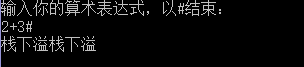2016-05-12 15:47

# C语言 为何总是提示“栈下溢”？

1``````#include <stdio.h>
#include <stdlib.h>
#include <string.h>
#include <malloc.h>
#include <conio.h>
#include <ctype.h>
#define maxsize 50

typedef char datatype;
typedef struct
{
int data[maxsize];
int Top;
} SeqStack;

void InitStack(SeqStack*&S)
{
S = (SeqStack*)malloc(sizeof(SeqStack));
S->Top = 0;
}

int push(SeqStack*s,datatype e)  //入栈函数
{
if (s->Top>=maxsize - 1)
{
printf("栈上溢");
return 0;
}
else
{
s->data[++s->Top] = e;
return 1;
}
}

char pop(SeqStack*s,datatype&e)  //出栈函数
{
if (s->Top<=0)
{
printf("栈下溢");
return 0;
}
else
{
return e = s->data[s->Top--];
}
}

char GetTop(SeqStack*s,datatype&e)  //取栈顶元素函数
{
if (s->Top<=0)
{
printf("栈下溢");
return 0;
}
else
{
e = s->data[s->Top];
return 1;
}
}

{
{
case '#':
return '>';
case '+':
case '-':
if (top == '#')
{
return '<';
}
else
return '>';
case '*':
case '/':
if (top == '+'||top == '-'||top == '#')
{
return '<';
}
else
return '>';
}
}

char operate(char n1,char op,char n2)  //运算函数
{
int sum;char s;
switch (op)
{
case '+':
sum = (n1 - 48) + (n2 - 48);
case '-':
sum = (n1 - 48) - (n2 - 48);
case '*':
sum = (n1 - 48) * (n2 - 48);
case '/':
sum = (n1 - 48) / (n2 - 48);
}
s = (char)sum;
return s;
}

int EvaluateExpression()
{
SeqStack *OPTR;
SeqStack *OPND;
InitStack(OPTR);
InitStack(OPND);
char ch;char top;char poper;
push (OPTR,'#');
while (((ch = getchar()) != '#')||GetTop(OPTR,top) != '#')
{
if (ch != '+'&&ch != '-'&&ch != '*'&&ch != '/'&&ch != '('&&ch !=')')
{
push (OPND,ch);
}
else
{
switch (precede(GetTop(OPTR,top),ch))  //判断优先级
{
case '<':
push (OPTR,ch);
break;
case '>':
char op = pop (OPTR,poper);
char b = pop (OPND,poper);
char a = pop (OPND,poper);
push (OPND,operate(a,op,b));
break;
}
}
}
int result = (int)GetTop(OPND,top);
return result;
}

int main()
{
int result;
printf("输入你的算术表达式，以#结束：\n");
result=EvaluateExpression();
printf("结果是 :%d\n",result);
return 0;
}
``````
• 点赞
• 写回答
• 关注问题
• 收藏
• 复制链接分享
• 邀请回答

#### 2条回答

• 帮楼主调试了下发现，假设你输入2+3#
在第二次循环，即处理+的时候,会跳入case '>':分支，然后会pop两次，但此时其实栈里面只有一个数字2
char b = pop (OPND,poper);
char a = pop (OPND,poper);
执行完char a = pop (OPND,poper);自然就显示栈下溢
之后的这句push (OPND,operate(a,op,b));也不对，你传入的op此时是#
里面的operate函数不会进入任何一个case,而是直接执行 s = (char)sum;

单步调试和设断点调试（VS IDE中编译连接通过以后，按F10或F11键单步执行，按Shift+F11退出当前函数；在某行按F9设断点后按F5执行停在该断点处。）是程序员必须掌握的技能之一。

点赞 评论 复制链接分享
• 不知道，帮忙顶顶。。。。。。。。。。。

点赞 评论 复制链接分享